# Exam-Style Questions.

## Problems adapted from questions set for previous Mathematics exams.

### 1.

GCSE Higher

The points B, C, D, E and F are on a circle. DAE and CAB are straight lines and AB= BE.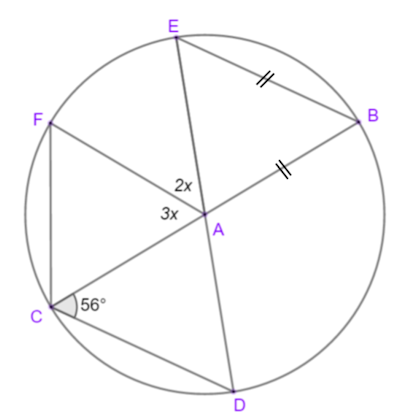Calculate the value of $$x$$.

### 2.

GCSE Higher

B, C, D and E are points on the circumference of a circle, centre O.

BD is a diameter of the circle and the diagram is not drawn to scale.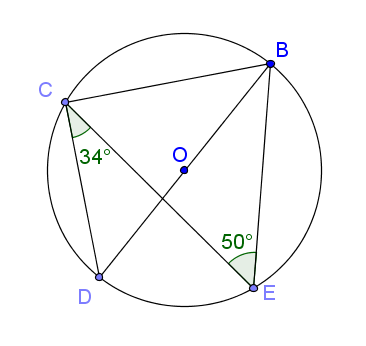(a) Find the size of angle DBE and give a reason for your answer.

(b) Find the size of angle DBC and give a reason for your answer.

### 3.

IGCSE Extended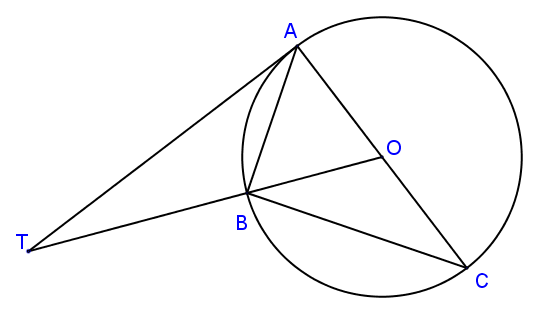In the diagram above, not drawn to scale, A, B and C are points on a circle, centre O. TA is a tangent to the circle at A and OBT is a straight line. AC is a diameter and angle OTA = 28°.

Calculate

(a) angle AOT

(b) angle ACB

(c) angle ABT

### 4.

GCSE Higher

(a) Find $$x$$, the size of angle ABC if B, C and D are all points on the circle to which AB is a tangent. The diagram is not drawn to scale.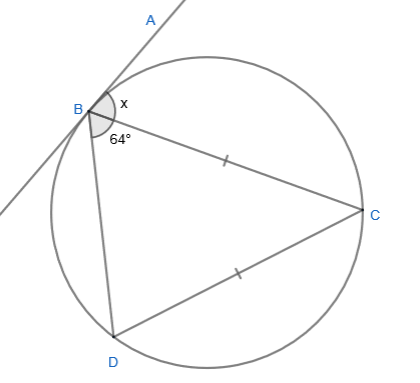### 5.

GCSE Higher

The diagram, not drawn to scale, shows chords joining points on the circumference of a circle. Prove that triangle ADE is similar to triangle BCE.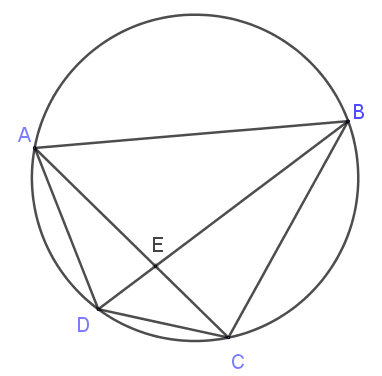### 6.

IGCSE Extended

The diagrams are not drawn to scale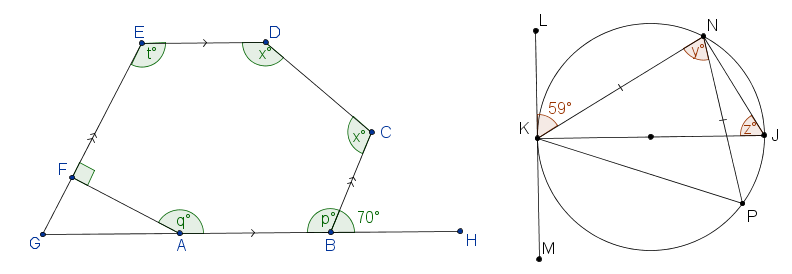ABCDEF is a hexagon with AB parallel to ED and BC parallel to FE. GFE and GABH are straight lines. Angle CBH = 70o and angle EFA = 90o. Calculate the values of: (a) $$p$$; (b) $$q$$; (c) $$t$$; (d) $$x$$. K, P, J and N are points on a circle and KN = NP. KJ is a diameter and LKM is the tangent to the circle at K. Angle LKN = 59o. Find the values of: (e) $$y$$; (f) $$z$$.

The exam-style questions appearing on this site are based on those set in previous examinations (or sample assessment papers for future examinations) by the major examination boards. The wording, diagrams and figures used in these questions have been changed from the originals so that students can have fresh, relevant problem solving practice even if they have previously worked through the related exam paper.

The solutions to the questions on this website are only available to those who have a Transum Subscription.

Exam-Style Questions Main Page

Search for exam-style questions containing a particular word or phrase:

To search the entire Transum website use the search box in the grey area below.